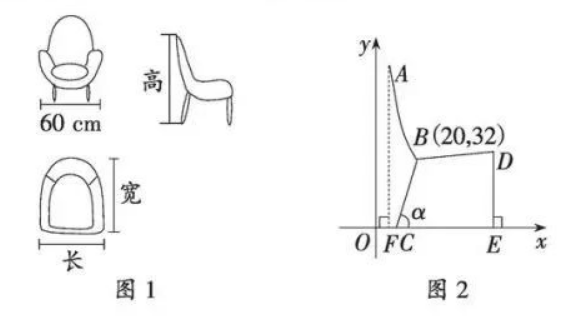(1) 请求出反比例函数表达式和一次函数表达式 (不要求写 $x$ 的取值范围);
(2) 过点 $A$ 向 $x$ 轴作垂线, 交 $x$ 轴于点 $F$, 已知 $C F=4 \mathrm{~cm}, D E=40 \mathrm{~cm}, \tan \alpha=4$, 设计师想用一 个长方体箱子将沙发放进去, 则这个长方体箱子长、宽、高至少分别是多少?